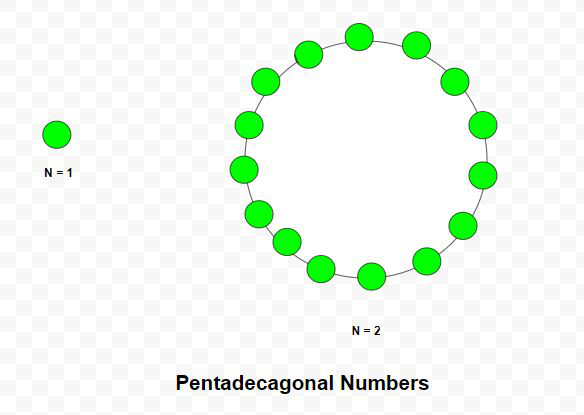Related Articles

• Last Updated : 17 Mar, 2021

Given a number N, the task is to find the Nth Pentadecagonal number.

A Pentadecagonal number is a figurate number that extends the concept of triangular and square numbers to the pentadecagon(a 15-sided polygon). The Nth pentadecagonal number counts the number of dots in a pattern of N nested pentadecagons, all sharing a common corner, where the ith tridecagon in the pattern has sides made of ‘i’ dots spaced one unit apart from each other. The first few Pentadecagonal numbers are 1, 15, 42, 82, 135, 201, 280 …

Examples:

Input: N = 2
Output: 15
Explanation:
The second Pentadecagonal number is 15.
Input: N = 6
Output: 201Approach: The Nth Pentadecagonal number is given by the formula:

``

Below is the implementation of the above approach:

## C++

 `// C++ program to find Nth``// Pentadecagonal number``#include ``using` `namespace` `std;` `// Function to find N-th``// Pentadecagonal number``int` `Pentadecagonal_num(``int` `n)``{``    ``// Formula to calculate nth``    ``// Pentadecagonal number``    ``return` `(13 * n * n - 11 * n) / 2;``}` `// Driver code``int` `main()``{``    ``int` `n = 3;``    ``cout << Pentadecagonal_num(n) << endl;``   ` `    ``n = 10;``    ``cout << Pentadecagonal_num(n) << endl;` `    ``return` `0;``}`

## Java

 `// Java program to find Nth``// pentadecagonal number``import` `java.io.*;``import` `java.util.*;` `class` `GFG{``    ` `// Function to find N-th``// pentadecagonal number``static` `int` `Pentadecagonal_num(``int` `n)``{``    ` `    ``// Formula to calculate nth``    ``// Pentadecagonal number``    ``return` `(``13` `* n * n - ``11` `* n) / ``2``;``}``    ` `// Driver code``public` `static` `void` `main(String[] args)``{``    ``int` `n = ``3``;``    ``System.out.println(Pentadecagonal_num(n));``    ` `    ``n = ``10``;``    ``System.out.println(Pentadecagonal_num(n));``}``}` `// This code is contributed by coder001`

## Python3

 `# Python3 program to find Nth``# pentadecagonal number` `# Function to find N-th``# pentadecagonal number``def` `Pentadecagonal_num(n):``    ` `    ``# Formula to calculate nth``    ``# pentadecagonal number``    ``return` `(``13` `*` `n ``*` `n ``-` `11` `*` `n) ``/` `2` `# Driver code   ``n ``=` `3``print``(``int``(Pentadecagonal_num(n)))` `n ``=` `10``print``(``int``(Pentadecagonal_num(n)))` `# This code is contributed by divyeshrabadiya07`

## C#

 `// C# program to find Nth``// pentadecagonal number``using` `System;``class` `GFG{``    ` `// Function to find N-th``// pentadecagonal number``static` `int` `Pentadecagonal_num(``int` `n)``{``    ` `    ``// Formula to calculate nth``    ``// Pentadecagonal number``    ``return` `(13 * n * n - 11 * n) / 2;``}``    ` `// Driver code``public` `static` `void` `Main(``string``[] args)``{``    ``int` `n = 3;``    ``Console.Write(Pentadecagonal_num(n) + ``"\n"``);``    ` `    ``n = 10;``    ``Console.Write(Pentadecagonal_num(n) + ``"\n"``);``}``}` `// This code is contributed by rutvik_56`

## Javascript

 ``
Output:
```42
595```

Attention reader! Don’t stop learning now. Get hold of all the important DSA concepts with the DSA Self Paced Course at a student-friendly price and become industry ready.  To complete your preparation from learning a language to DS Algo and many more,  please refer Complete Interview Preparation Course.

In case you wish to attend live classes with experts, please refer DSA Live Classes for Working Professionals and Competitive Programming Live for Students.

My Personal Notes arrow_drop_up